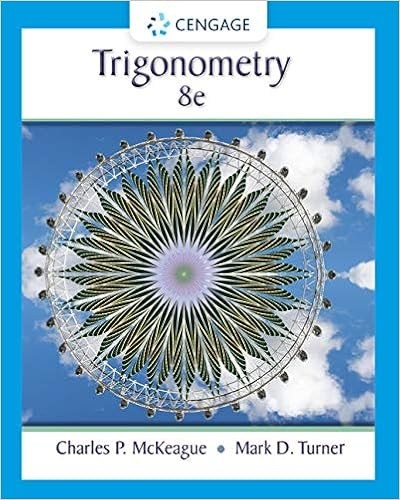# E x a m p l e 1 u calculator close up v to evaluate

• Assessment
• tblacksh
• 76
• 100% (1) 1 out of 1 people found this document helpful

This preview shows page 2 - 5 out of 76 pages.

##### We have textbook solutions for you!
The document you are viewing contains questions related to this textbook.The document you are viewing contains questions related to this textbook.
Chapter 7 / Exercise 22
Trigonometry
McKeague/TurnerExpert Verified
notation as we did for polynomials in Chapter 5. E X A M P L E 1 U Calculator Close-Up V To evaluate the rational expression in Example 1(a) with a calculator, first use Y = to define the rational expres­ sion. Be sure to enclose both numera­ tor and denominator in parentheses. Then find y 1 ( - 3). Evaluating a rational expression a) Find the value of 4 x x + - 2 1 for x = - 3. b) If R ( x ) = 3 2 x x + - 2 1 , find R (4). Solution a) To find the value of 4 x x + - 2 1 for x = - 3, replace x by - 3 in the rational expression: 4( - - 3 3 ) + - 2 1 = - - 1 1 3 = 13 So the value of the rational expression is 13. The Calculator Close-Up shows how to evaluate the expression with a graphing calculator using a variable. With a scientific or graphing calculator you could also evaluate the expression by entering (4( - 3) - 1) / ( - 3 + 2). Be sure to enclose the numerator and denominator in parentheses. b) R (4) is the value of the rational expression when x = 4. To find R (4), replace x by 4 in R ( x ) = 3 2 x x + - 2 1 : R ( 4 ) = 3 2 ( ( 4 4 ) ) + - 2 1 R (4) = 1 7 4 = 2 So the value of the rational expression is 2 when x = 4, or R (4) = 2 (read “ R of 4 is 2”). Now do Exercises 1–6
##### We have textbook solutions for you!
The document you are viewing contains questions related to this textbook.The document you are viewing contains questions related to this textbook.
Chapter 7 / Exercise 22
Trigonometry
McKeague/TurnerExpert Verified
6-3 6.1 Reducing Rational Expressions 383 An expression such as 5 is undefined because the definition of rational numbers 0 does not allow zero in the denominator. When a variable occurs in a denominator, any real number can be used for the variable except numbers that make the expression undefined. E X A M P L E 2 Ruling out values for x Which numbers cannot be used in place of x in each rational expression? a) x x 2 + - 8 1 b) 2 x x + + 2 1 c) x x 2 + - 5 4 Solution a) The denominator is 0 if x + 8 = 0, or x = - 8. So - 8 cannot be used in place of x . (All real numbers except - 8 can be used in place of x .) b) The denominator is zero if 2 x + 1 = 0, or x = - 1 2 . So we cannot use - 1 2 in place of x. ( All real numbers except - 1 2 can be used in place of x. ) c) The denominator is zero if x 2 - 4 = 0. Solve this equation: x 2 - 4 = 0 ( x - 2)( x + 2) = 0 Factor. x - 2 = 0 or x + 2 = 0 Zero factor property x = 2 or x = - 2 So 2 and - 2 cannot be used in place of x . (All real numbers except 2 and - 2 can be used in place of x .) Now do Exercises 7–14 E X A M P L E In Example 2 we determined the real numbers that could not be used in place of the variable in a rational expression. The domain of any algebraic expression in one variable is the set of all real numbers that can be used in place of the variable. For rational expressions, the domain must exclude any real numbers that cause the denom­ inator to be zero. Domain Find the domain of each expression. 2 x - 9 x x - 5 a) b) 2 c) x + 3 x - x - 6 4 Solution a) The denominator is 0 if x + 3 = 0, or x = - 3. So - 3 can’t be used for x. The domain is the set of all real numbers except - 3, which is written in set notation as { x 1 x e - 3}. b) The denominator is 0 if x 2 - x - 6 = 0: 2 x - x - 6 = 0 ( x - 3)( x + 2) = 0 x - 3 = 0 or x + 2 = 0 x = 3 or x = - 2 So - 2 and 3 can’t be used in place of x . The domain is the set of all real numbers except - 2 and 3, which is written as { x 1 x e - 2 and x e 3}.
384 Chapter 6 Rational Expressions 6-4 c) Since the denominator is 4, the denominator can’t be 0 no matter what number is used for x . The domain is the set of all real numbers, R.
•••